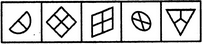# Non Verbal Reasoning - Classification - Discussion

### Discussion :: Classification - Section 1 (Q.No.8)

In each problem, out of the five figures marked (1), (2), (3), (4) and (5), four are similar in a certain manner. However, one figure is not like the other four. Choose the figure which is different from the rest.

8.

Choose the figure which is different from the rest.(1)     (2)     (3)     (4)     (5)

 [A]. 1 [B]. 2 [C]. 3 [D]. 4 [E]. 5

Explanation:

All other figures are divided into equal parts.

 Barbie said: (Jul 2, 2011) Yes ans A is correct. But 5 is divided into 3 equal parts. So, I think this is wrong.

 Chinmay said: (Feb 16, 2012) I think all parts divided are of same size except 4. So answer is rite.

 Wasim said: (Apr 22, 2013) Let me clear this the answer is absolutely right but conclusion is false. Have a look at 3rd fig. That fig is not divided into equal parts look closely. The answer is 4 Because all figs having their corners but have a close look at 4th fig it does not have any corner. So the answer is 4 and this is the solution.

 Partha Ghosh said: (Jul 17, 2015) I think all are divided into equal parts except 5.

 Teja said: (Sep 11, 2015) 4th is also divided into equal parts, except the 5th.

 Sanjaysinh said: (Dec 26, 2015) But in question 3 also at some angle so not divided equally.

 Roshan Roy said: (Mar 10, 2016) Figure (4) is not divided into equal parts unlike figure (1), (2), (3), (5). The lower left quarter and higher right quarter is bigger than the higher left quarter and lower right quarter.

 Debdeep Khan said: (Sep 21, 2016) The answer should be A.

 Tasbi said: (Jan 12, 2017) Not getting this, Please explain briefly.

 Sumit said: (Mar 11, 2017) Answer 5 is also not divided in equal part.

 Rishabh Agrawal said: (Mar 21, 2017) I think the answer is A because 4 is looking like the picture from another angle and its look like equal.

 Prabhjot Kaur said: (May 21, 2017) Because Figure 4 has no corners. Am I right?

 Yogesh said: (May 30, 2017) Yeah, right @Prabhjot.

 Cherry said: (Jul 13, 2017) I think the answer is (1) because the shape is irregular, unlike the other shapes.

 Yamini Gautam said: (Aug 21, 2017) The answer is (4). Since the circle is not divided into 4 equal parts (2 parts are of 120 degree sectors and 2 others are of 60 degree sectors) whereas all the others are equally divided including (5).

 Sai Girish said: (Oct 12, 2017) I think the answer is 5. It is not divided equally.

 Sam said: (Oct 26, 2017) 5 is correct Answer as the triangle has no lines of symmetry so how it's equally divided.

 Neil said: (Oct 28, 2017) I think answer A is correct.

 Neeraja said: (Nov 14, 2017) Answer is 4, in all figures every diagram is having corners except fig 4 doesn't have any corner.

 Rupesh Kumar said: (Oct 12, 2018) I think Answer A is correct.

 Anoms said: (Mar 31, 2019) 5 is the correct answer. For isometric projection circle always looks like an ellipse.

 Ramu said: (Apr 17, 2019) The Answer may be 5 because all are divided into four parts in 1 also it is semi-circle divided into two parts if it is circle 4. In 5, it is only 3.

 Sma said: (Jun 12, 2019) The answer is 4. As it looks like it is divided into equal portions.

 Saipranav said: (Oct 15, 2019) I think 5 is not equally divided so both 1&5are wrong.

 Ayush said: (Jun 7, 2020) According to me, Answer should be "E" because other than that all others are dividing into equal parts.

 Vishnu said: (Jun 17, 2020) Yes, I too agree, It's option A.

 Janhavi said: (Aug 15, 2021) Why 5 is not the answer? All other figures are divided in an even number of parts and the 5th is divided into an odd number of parts.